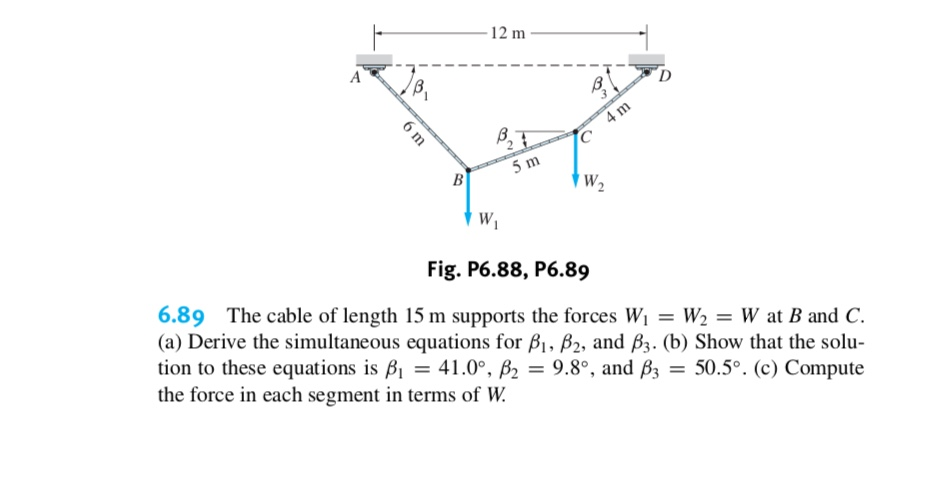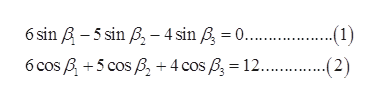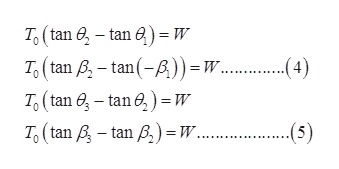# 12 m4 m5 mBW2Fig. P6.88, P6.896.89 The cable of length 15 m supports the forces Wi = W2 = W at B and C(a) Derive the simultaneous equations for B, B2, and B3. (b) Show that the solution to these equations is B = 41.0°, B2 = 9.8°, and B3the force in each segment in terms of W50.5°. (c) Compute6 m

Question
3 viewshelp_outlineImage Transcriptionclose12 m 4 m 5 m B W2 Fig. P6.88, P6.89 6.89 The cable of length 15 m supports the forces Wi = W2 = W at B and C (a) Derive the simultaneous equations for B, B2, and B3. (b) Show that the solu tion to these equations is B = 41.0°, B2 = 9.8°, and B3 the force in each segment in terms of W 50.5°. (c) Compute 6 m fullscreen
check_circle

Step 1

From geometry resolve the length of cable in vertical and horizontal direction;help_outlineImage Transcriptionclose6 sin A-5 sin B-4 sin B 0 ) ...1) --2) 6 cosB5 cos +4 cos B 12. fullscreen
Step 2

Express the total number of independent equilibrium Equation for a cable with n segments as follows:

Step 3

Apply Equation (3) in Joint B and C.

...help_outlineImage TranscriptioncloseTo(tan -tan )=W 4 T(tan B- tan(-A)) = .. To (tan e-tan e) =W To(tan -tan A)W.... (5) fullscreen

### Want to see the full answer?

See Solution

#### Want to see this answer and more?

Solutions are written by subject experts who are available 24/7. Questions are typically answered within 1 hour.*

See Solution
*Response times may vary by subject and question.
Tagged in

### Solid Mechanics